﻿ Non-Scattering Photon Electron Interaction

Non-Scattering Photon Electron Interaction

Zhiliang Cao, Henry Gu CaoOPEN ACCESSPEER-REVIEWED

Non-Scattering Photon Electron Interaction

Zhiliang Cao1,, Henry Gu Cao2

1J.P.Morgan Chase

2Deerfield High School, Deerfield

Abstract

We propose a new model of the interaction between photons and electrons. During this interaction, the photon’s direction of movement does not change. The electron’s velocity along the photon’s direction of movement is close to c/2 in order to allow the interaction to proceed. During the photon and electron energy exchange process, when an electron’s speed is less than 0.7071c and greater than 0.5c, the photon will lose energy while the electron will gain energy; when an electron’s speed is more than 0.7071c, the photon will gain energy while the electron loses energy. Three physical experiments are proposed. In the first experiment, the electron speed is set to 0.6c and a red-shift is expected to occur. In the second experiment, the electron speed is set to 0.8c and a blue-shift is expected to occur. In the third experiment, the electron speed is set to 0.7071c or 0.4c. The theory predicts that there won’t be a photon wavelength change.

At a glance: Figures

12
Prev Next

• Cao, Zhiliang, and Henry Gu Cao. "Non-Scattering Photon Electron Interaction." Physics and Materials Chemistry 1.2 (2013): 9-12.
• Cao, Z. , & Cao, H. G. (2013). Non-Scattering Photon Electron Interaction. Physics and Materials Chemistry, 1(2), 9-12.
• Cao, Zhiliang, and Henry Gu Cao. "Non-Scattering Photon Electron Interaction." Physics and Materials Chemistry 1, no. 2 (2013): 9-12.

 Import into BibTeX Import into EndNote Import into RefMan Import into RefWorks

1. Introduction

A photon is an electromagnetic wave. This paper will explain a new interaction model in which the photon and electron can exchange energy and moving momentum during collision without changing the photon’s direction. The proposed model is different from Campton Scattering (e.g. ). In Campton Scattering, the initial electron momentum can be ignored. But in this case, the initial electron momentum is large and can’t be ignored. Also, its momentum change is relatively small. Therefore relativity effects (e.g. [2-10]) will be considered, but energy changes as a result of relativity effects will be ignored.

2. Interaction

Assume that M is the mass of an electron, m is the portion of a photon’s loss or gain of mass during the interaction; Vx is the electron’s initial speed perpendicular to the photon’s moving direction; Vy is the electron’s initial speed parallel to the photon moving direction; Vc is the change in Vy of the electron after the interaction. The electron kinetic energy before the interaction is: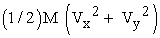The electron moving and spinning kinetic energy (with factor of a) after the interaction is: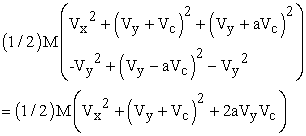m is the amount of a photon’s mass lost or gained during the interaction. Therefore the energy change is:

mc2

The following energy conservation and momentum conservation equations can be deducted:Combining the two equations,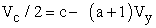(1)

Since Vc is small, from formula (1),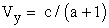(2)

When electron losses energy, a < 1.

2.1. Impact Tube

The wavelength of an electron is 1.22 * 10-12m. It is much less than the ultraviolet light’s 10-8m wavelength. A free electron can impact part of a photon (Figure 1). This is unlike the electron inside the atom, which has complex waves among which a photon may be able to find a longer wave to interact with.

Therefore, the electron limits its impact on the photon by its wave length in circular form (Figure 2).

For the fixed collision angle during the electron and photon collision, the track of the electron forms a tube (Figure 1).

Assume that the wavelength of photon is. Then the radius of the impact tube is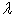and the possibility of the collision is: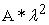Whereas A is an unknown constant.

Since the impact tube’s cross section is fixed, the energy change of the photon relative to the total photon energy is the volume of tube divided by the volume of photon, or:Whereas B is an unknown constant.

The average energy change rate of the photon is:Whereas K is an unknown constant.

Therefore, regardless of the photon’s wavelength, the average percent to the original photon energy impacted is the same.

2.2. Crossing Speed

Vx in Figure 1 is called the crossing speed. It determines the angle of the impacted tube of the photon. Assume Vy=c/2. The wavelength of photon is. Collision duration is t: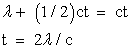(3)

The time of the electron and photon interaction can be twice the photon’s period of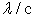. When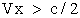, the electron can cross from one side of photon to the other. The total speed of the electron is:(4)

When the speed of electron is greater than 0.7071c, the electron starts to release energy to the photon, causing photon to blue-shift.

2.3. Blue-Shift

When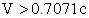, the photon mass increment follows (2).

In this case, the increased mass of m may have a larger unknown range.

3. Proposals for Experiments

3.1. Red-Shifting Case Formula

The electron has a charge of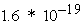coulombs. 0.00016A of electron flow has 1015 electrons per second. At speed of c/2, the number of electrons per half meter is.

The proper electron traveling speed is an important factor for positive test results.

Assume that a helium neon laser of 632.8 nm is used. The mass of a photon is: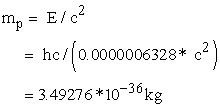Rest mass of an electron:When travelling at c/2, the mass of an electron is: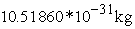From formula (3),The estimated maximum lost mass per collision, assuming that impact tube cross section radius is the electron wavelength, is:In other words, if 127749.7212425v is used, the gained voltage of the electron is 1.405µV. Fine voltage adjustment is critical to the success of the tests.

For 0.0016 A of electron flow in the ideal confined space in one micron diameter, the energy change factor is: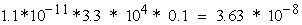If the electron pulses for 10 ns in one second intervals, the same power can generate 108 times of the original intensity. Energy change factor becomes: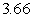3.2. Red-Shift Experiment Proposal

This experiment involves a confined electron and laser beam in a one meter long and one micro meter diameter tunnel. Both the electron and laser beam will pulse for 10ns in 1 second intervals. The electron emitter’s power is 204.4 W.

Use 127749.7212425v to make the electron travel at speed of 0.6c while the angle of the photon off the tube’s wall 10°, 20°, 30°, 40°, 50°, 60°, 70°, and 80°.

Be careful to make fine adjustments of the electron’s voltage and try to record any red-shifting of the laser beam.

3.2. Blue-Shift Experiment Proposal

Use the same device as above. The speed of electron is 0.8c (148.69783883654v) and angle of photon 10°, 20°, 30°, 40°, 50°, 60°, 70°, and 80°.

3.3. No Wavelength Change Experiment Proposal

When the electron’s speed is 0.4c or 0.7071c, there will be no photon wavelength change detected.

The following results are predicted for each successful energy exchange instance:

4. Feasibility of the Experiments

The electron microscope (e.g. [12-34]) uses a focused beam of high-energy electrons to produce a variety of signals at the surface of a solid specimen. The diameter of the focused electron beam can be less than one nanometer.

4.1. Overall Design

Some small modifications to existing electron microscopes can be used to create the following design of the equipment of the experiment:

4.2. Feasibility

The above design uses an electron microscope and existing spectrograph technology. The cost can be as low as building an electron microscope.

The reason for using a smaller tunnel is to use a weaker electron beam for safety considerations. If the one micro meter diameter tunnel can not be built, then a wider tunnel can be used. To have same effects, the tunnel must be 5 µm diameter and it should have a one micro meter hole at the entrance of the tube and a one micro meter hole at the exit of the tube. The electron beam needs to last for 250 ns instead. The power will be increased to 5000w. If 5000w is not safe, then the power should be reduced. But the effects will still be detectable. The adjustable laser source angle controller should also be easy to build. Overall, the experiment is feasible.

References

  Compton, Arthur H. (May 1923). "A Quantum Theory of the Scattering of X-Rays by Light Elements". Physical Review 21 (5): 483-502.In article CrossRef  Einstein A. (1916 (translation 1920)), Relativity: The Special and General Theory, New York: H. Holt and Company.In article CrossRef  Planck, Max (1906), "The Measurements of Kaufmann on the Deflectability of β-Rays in their Importance for the Dynamics of the Electrons", Physikalische Zeitschrift 7: 753-761.In article  Miller, Arthur I. (1981), Albert Einstein's special theory of relativity. Emergence (1905) and early interpretation (1905-1911), Reading: Addison–Wesley.In article  Will, Clifford M (August 1, 2010). "Relativity". Grolier Multimedia Encyclopedia. Retrieved 2010-08-01.In article  Will, Clifford M (August 1, 2010). "Space-Time Continuum". Grolier Multimedia Encyclopedia. Retrieved 2010-08-01.In article  Will, Clifford M (August 1, 2010). "Fitzgerald–Lorentz contraction". Grolier Multimedia Encyclopedia. Retrieved 2010-08-01.In article  Einstein, Albert (November 28, 1919). "Time, Space, and Gravitation". The Times.In article  Feynman, Richard Phillips; Morínigo, Fernando B.; Wagner, William; Pines, David; Hatfield, Brian (2002). Feynman Lectures on Gravitation. West view Press. p. 68. Lecture 5.In article  Roberts, T; Schleif, S; Dlugosz, JM (ed.) (20 07). "What is the experimental basis of Special Relativity?". Usenet Physics FAQ. University of California, Riverside. Retrieved 2010-10-31.In article  Livingston, M. S.; Blewett, J. (1962). Particle Accelerators. New York: McGraw-Hill.In article PubMed  Karimi E, Marucci L, Grillo V and Santamato E 2012 Phys. Rev Lett 108 044801.In article CrossRef PubMed  Uchida M and Tonomura A 2010 Nature 737 464.In article  Verbeeck J, Tian H and Schattschneider P. 2010 Nature 467 301.In article CrossRef PubMed  McMorran B J, Agrawal A, Anderson I M, Herzing A A, Lezec H J, McClelland J J and Unguris J 2011 Science 331 192.In article CrossRef PubMed  Cowley J M and Moodie A F 1957 Acta Crystallographica 10 609.In article CrossRef  Pozzi G 1995 Advances in Imaging and Electron Physics 93 173.In article CrossRef  Ehremberg W and Siday, R E 1949 Proc. Phys. Soc. B 62 8.In article CrossRef  Aharonov Y and Bohm D 1959 Phys. Rev. 115 485.In article CrossRef  Jagannathan R. 1990 Phys. Rev. A 42 6674.In article CrossRef PubMed  Rother A and Scheerschmidt K 2009 Ultramicroscopy 109 154.In article CrossRef PubMed  Pozzi G 1989 Ultramicroscopy 30 417.In article CrossRef  Fujiwara K. 1961 J. Phys. Soc. Jpn. 16 2226.In article CrossRef  Jagannathan R 1990 Phys. Rev. A 42 6674.In article CrossRef PubMed  Cohen-Tannoudji C, Diu B and Laloe F, 1977 Quantum Mechanics (Wiley and Hermann, Paris).In article  Kirkland E J 2010 Advanced Computing in Electron Microscopy (Springer).In article CrossRef  Grillo V and Rotunno E 2013. UltramicroscopyIn article  (http://dx.doi.org/10.1016/j.ultramic.2012.10.016).In article  Rose H and Wan W, Aberration Correction in Electron Microscopy, presented at Proceedings of 2005 Particle Accelerator Conference, (Knoxville, Tennessee) (unpublished).In article CrossRef  Hasselbach F. 2010 Rep. Prog. Phys. 73 016101.In article CrossRef  Karimi E, Piccirillo B, Nagali E, Marrucci L and Santamato E 2009 Appl. Phys. Lett. 94 231124.In article CrossRef PubMed  Nicklaus M and Hasselbach F. 1993 Physical Review A 48 152In article CrossRef PubMed  Krivanek, O. and al. 2009 Phil. Trans. R. Soc. A 367 3683.In article  Verbeek J and Schattschneider P 2011 Ultramicroscopy 111, 1461 (2011).In article  Baartman R 1997 presented at Proc. Particle Accelerator Conference, Vancouver, British Columbia, Canada, (unpublished).In article